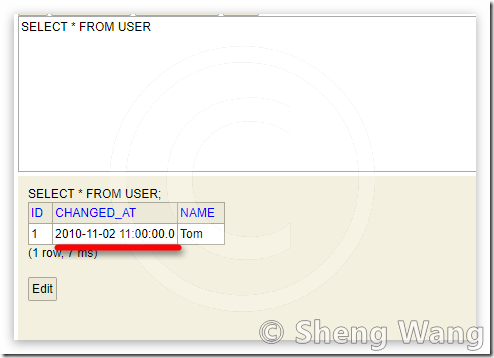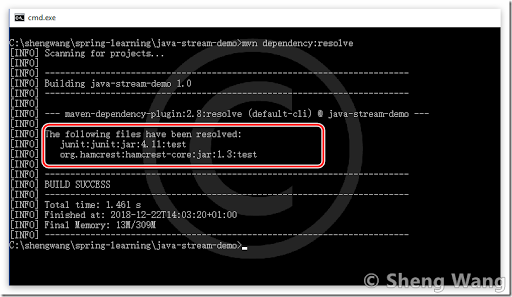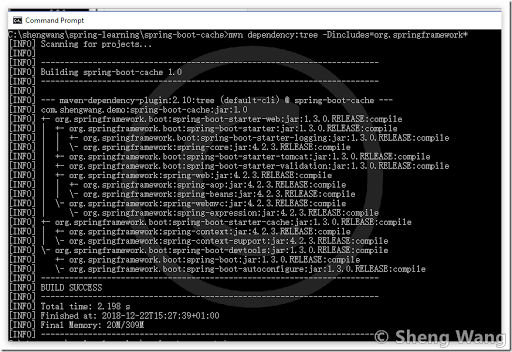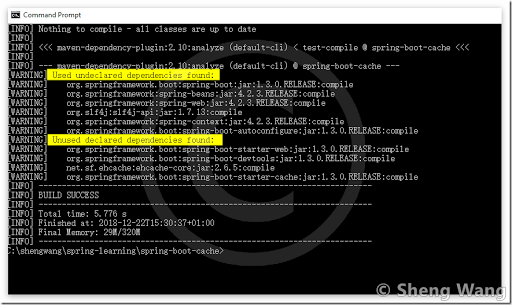# Code Breeze !

## Pitfall when using hibernate + date in entity

It’s a frequently encountered pitfall when using hibernate, also has entity with properties type defined as java.util.Date.

In short, although the entity’s property is defined as java.util.Date in entity class, but at runtime hibernate will never set it as java.util.Date, but java.sql.Timestamp!

Since java.util.Date is the parent class of java.sql.Timestamp, so in your code whenever treat this field as java.util.Date, there is no exeption at compilation or runtime. But the unexpected result often come when the eqals operation involved.

An instance of java.sql.Timestamp will NEVER equal to any instance of java.util.Date! (caused by Timestampl’s impl of equals method, see javadoc of Timestamp), even logically they represent the exactly time.

The following example shows you how this pitfall looks like.

## Entity class

Suppose we have a entity class ‘User’.

```@Entity
@Table(name = "user")
public class User {
@Id
private Long id;
private String name;
@Column(name = "changed_at")
private Date changedAt;

// setter getter omitted
}```

There is a field “changedAt” defined as time java.util.Date.

## Database

In database, we have following data## code to show the pitfall

Let’s run the following code.

```        DateFormat df = new SimpleDateFormat("yyyy-MM-dd HH:mm:ss");
Date a = df.parse("2010-11-02 11:00:00");       // a is instance of Date
Date b = userRepository.findById(id).get().getChangedAt();  // b is actually a instance of Timestamp

System.out.println("a.getTime() == b.getTime() is " + (a.getTime() == b.getTime()));
System.out.println("a.equals(b) is " + a.equals(b));
System.out.println("b.equals(a) is " + b.equals(a));```

Although the variable is also defined a ‘Date’ but thanks to hibernate,  at runtime it’s an instance of Timestamp

Then we have the following output

```a.getTime() == b.getTime() is true
a.equals(b) is true
b.equals(a) is false```

As you can see, even a and b represent the same logic time, the equals result are different.  You many wonder why b.equals (a) is false, here is the reason.

```    public boolean equals(java.lang.Object ts) {
if (ts instanceof Timestamp) {
return this.equals((Timestamp)ts);
} else {
return false;
}
}```

Above is the equals() impl of class java.sql.Timestamp. Caused by the instanceof check, a instance of Timestamp never equals to instance of Date.

## Conclusion

• When work with hibernate and entity with date, always remember they are not real ‘Date’, but ‘Timestamp’,
• Always use Data instance’s equals() methods

## Several useful cases for maven plugin “dependency”

The maven dependency plugin is very handy when trying to deal with following questions:

• What are the external artifacts involved in a maven project. (dependency:resolve)
• Find out how an artifact is introduced into a project. (dependency:tree)
• In a multi modules project, how these modules denpend on each other? (dependency:tree –Dincludes=com.mypackage.*)
• Find out unnecessary dependencies in a maven project. (dependency:analyze)

To solve these puzzles, we need to know some typical usage of maven dependency plugin.

## 1. goal ‘resolve’

```#list all dependencis
mvn dependency:resolve ```

Output looks like below.## 2. goal ‘tree’

```# list dependencies tree.
# can use –Dincludes=[groupId]:[artifactId]:[type]:[version], can use wildcard *

# e.g  -Dincludes=org.springframework*,org.apache*    # list only package from spring and apache.

mvn dependency:tree  -Dincludes=org.springframework*```

Output looks like below.## 3. goal ‘analyze’

```# List possible inmproper dependency.
# 1.avoid ‘Used undeclared dependencies`
# 2.'Unused declared dependencies` are not 100% reliable, cause of <provide> or <optional>
#    need to check manually

mvn dependency:analyze```

Output looks like below.In above screenshot there are several suspicious dependencis may not be used at all. Then use “dependency:tree –Dincludes= org.springframework.boot:spring-boot-starter-web” to see how this dependency is introduced to the project.